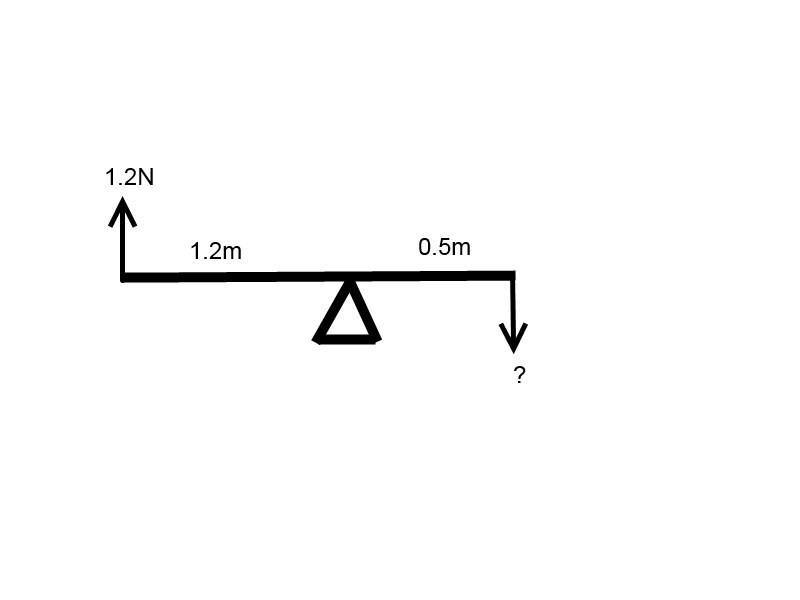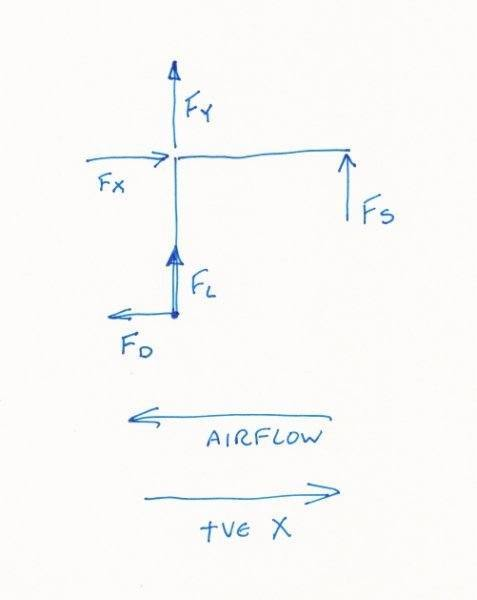# Work out force transmitted to sensor

Engineer101

## Homework Statement

There is a wind tunnel force balance allowing the
measurement of the drag force resultant at point A of a test object in the air
stream from an arm and lever system mounted on a pivoting point O and
pressing onto a force sensor at point B.

1) What will be magnitude of the force transmitted into the sensor at B if the air
flow results in creating a lift force of 1.75 N and a drag force of 1.20 N?

I couldn't paste in the picture it wouldn't let me. but there is a 90 degree lever with the first part of the lever being vertical in the direction of lift and is 1.2m long. There is then a pivot, O, and to the right of that at 90 degrees is a horizontal part of the lever and is 0.5m long. sensor B is underneath this part so that when drag occurs it will push on the lever and push down onto B.

2) Calculate the horizontal component Ox and vertical component, Oy of the transmitted force in pivoting point O.
(Consider the coordinate system used to be positive in the direction against the airflow for the x component, and positive in the upward direction for y)

Pythagoras?

## The Attempt at a Solution

question 1 - The sensor is used to measure lift so is the answer simply 1.20N?
I tried resolving the force and then did some moments but then realised I only need an answer in Newtons and I would get an answer in N/m.

question 2 - is it -1.20 since the force transmitted is in the opposite direction to airflow? and vertical component 0? I'm confused because the lengths of the lever arms seem to be irrelevant.

This is my first post, thank you for reading and helping if you can!
Thanks.

Last edited by a moderator:

Homework Helper
Gold Member
question 1 - The sensor is used to measure lift ...

Is it? The problem statement says..

There is a wind tunnel force balance allowing the
measurement of the drag force...

The answer isn't 1.2N. There are levers of different lengths involved.

Engineer101
Sorry I meant to say drag,
So how do you work it out then? Because why would you have a sensor that measures drag that doesn't read 1.2N?
Thank you!

Homework Helper
Gold Member
(For Q1) This diagram is effectively the same problem. Any easier to understand?Homework Helper
Gold Member
Because why would you have a sensor that measures drag that doesn't read 1.2N?

The question doesn't ask what the sensor displays it asks for the "force transmitted into the sensor"

Engineer101
(For Q1) This diagram is effectively the same problem. Any easier to understand?
View attachment 76779
Ah that is a bit easier, is it 0.5N then?

Homework Helper
Gold Member
Try again. Have a look at the length of the levers. Will the force be greater or less than 1.2N ?

Engineer101
Try again. Have a look at the length of the levers. Will the force be greater or less than 1.2N ?
Actually it would be more, but how do you do it without getting the answer in Newton Meter's? Is it 2.88N?
Thanks

Homework Helper
Gold Member
Correct.

Torque = force * distance
= 1.2N * 1.2M
= 1.44 NM

It's the same torque on the other arm so using..

Force = Torque/Distance

= 1.44NM / 0.5M
= 2.88N

Have a go at Q2. May help to add the 2.88N force to the diagram.

Engineer101
Correct.

Torque = force * distance
= 1.2N * 1.2M
= 1.44 NM

It's the same torque on the other arm so using..

Force = Torque/Distance

= 1.44NM / 0.5M
= 2.88N
Great thank you!
How about the x and y components? How would I go about solving that? Trig?

Homework Helper
Gold Member
Start with the original drawing in the link you posted and produce a free body diagram for the cranked beam.

a) the 2.88N force that the sensor puts on the beam.
b) arrows representing the x and y forces at the pivot.

The beam isn't accelerating (in any direction) so what does that mean for the net force on the beam (in any direction)?

PS: Remember the question says...

Consider the coordinate system used to be positive in the direction against the airflow for the x component, and positive in the upward direction for y

Last edited:
Engineer101
It is 0?
So you'll have the reaction force in the y direction?

Homework Helper
Gold Member
The net force in the horizontal (or vertical) direction will be zero because F=ma so if a = 0 then F=0.

But show your working. The recommended procedure for any similar problem is to:

Make the drawing
Define which direction(s) are positive
Label the forces
Write the equations
Solve any simultaneous equations (if necessary)
Substitute values

PS In most exams I've sat you get marks for getting the procedure right even if you make an arithmetic somewhere near the end. If you only give the answer and it's wrong due to a trivial error you get zip.

Engineer101
Thank you so much! That has helped lots thank you! Yeah we get that in the UK too, could get most the marks with all the wrong answers
So in the x direction it'll be -2.88N since it'll be in the opposite direction to air flow

Homework Helper
Gold Member
I'm also in the uk.

So in the x direction it'll be -2.88N

The sign is correct but how did you get the 2.88N part?

Homework Helper
Gold Member
Correction the sign is also wrong...

So in the x direction it'll be -2.88N since it'll be in the opposite direction to air flow

Consider the coordinate system used to be positive in the direction against the airflow

Last edited:
Engineer101
Because it is transferring 2.88N of force to the sensor, so if y is 0N, then the x component must be the remaining 2.88N?
Damn. All wrong

Homework Helper
Gold Member
Because it is transferring 2.88N of force to the sensor, so if y is 0N, then the x component must be the remaining 2.88N?

Err that sounds all mixed up.

Back in a moment.

Homework Helper
Gold Member
Here is my Free Body Diagram.

FD = Drag Force = -1.2N
FL = Lift Force = 1.75N
FS = Sensor force = 2.88N
FY = Y component of force at pivot
FX = X component of force at pivot

The drag force is to the left so that is also the direction of the airflow.

The problem says to assume:
X is "positive in the direction against the airflow" so +ve is to the right.
Y is "positive in the upward direction"

Have a look and then write an equation that sums all the horizontal forces to zero.Engineer101
I dont know what equations they could be though?
Force of drag - x component = 0? So x component = Fd?

Homework Helper
Gold Member
I would write..

FX + FD = 0
rearrange..
FX = - FD
Substitute for FD
FX = -(-1.2)
= 1.2N

Try it for the vertical.

Engineer101
Great thank you!
Fy+Force lift = 0
Fy=- Force lift
Fy= -1.75N
But it should be 0?

Homework Helper
Gold Member
You forgot the sensor force...

FY + FL + FS = 0
FY = - FL - FS
= -1.75 - 2.88
= -4.63N

Engineer101
Ah of course that's vertical as well,
I thought you said the y component was 0 as it wasn't accelerating though?

Homework Helper
Gold Member
I thought you said the y component was 0 as it wasn't accelerating though?

It's not the y component that's zero.

If an object isn't accelerating in any direction (including rotational acceleration) then

All the horizontal forces sum to zero
All the vertical forces sum to zero
All the rotational forces (aka torques) sum to zero

So vertically..
FY + FL + FS = 0

In some problems the forces aren't horizontal or vertical but at some angle (theta) so you have to break down those forces into horizontal and vertical components then sum them. This tends to gives you equations like..

Vertical..

F*Sin(theta) + some other forces = 0

Horizontal..

F*Cos(theta) + some other forces = 0

Homework Helper
Gold Member
You can have situations where an object is accelerating vertically but not horizontally. In which case the horizontal forces must sum to zero but the vertical ones won't.

Engineer101
That has helped me so much thank you!
I understand that now, I just have trouble figuring out where to begin.
This mechanical test is really hard, I actually got 100% in the electronics one believe it or not, and I don't like electronics at all.
Would it be okay to get some of your help on other questions I find tricky?
Thanks!

Homework Helper
Gold Member
Happy to help if I can but best post questions to the forum rather than me personally as I'm not always around.

PS: I have twins age 13 who have their own homework problems!

Engineer101
Happy to help if I can but best post questions to the forum rather than me personally as I'm not always around.

PS: I have twins age 13 who have their own homework problems!
Sure, that's fine help if you're around!
Thanks! They must get good grades haha

Homework Helper
Gold Member
Thought it might be worth checking that the torque really do sum to zero. If clockwise is +ve then...

Summing the torques about the pivot point gives..

(FD * 1.2) - (FS* 0.5) = ?
(1.2 * 1.2) - (2.88 * 0.5) = ?
1.44 - 1.44 = 0

So that's ok.

However if it's not accelerating in rotation you can actually sum the torques about any point and it will be zero. eg It doesn't have to be a real pivot.

Suppose we pick the sensor and sum the torque about that point. The equation is..

(FD*1.2) + (FL*0.5) +(FY*0.5) = ?

FX and FS don't feature because they pass through the point where the sensor is located (eg tangential distance = 0).

Substitute values..

(1.2*1.2) + (1.75*0.5) + (-4.63*0.5) = ?

1.44 + 0.875 - 2.315 = 0

This ability to pick any point about which to sum torques is handy. For example suppose there is an unknown force somewhere. If you pick the point where that force acts you eliminate it from the equation at a stroke.

Engineer101
We learnt about this in my lectures this semester and I couldn't visualise it too well, but you've helped me understand it a lot better thank you!